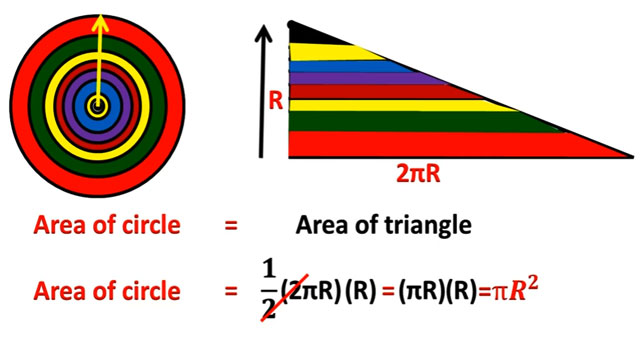Derivation of area of circle

# Derivation of area of circleThis construction video will provide brief information on the formula derivation toward circle area. To estimate the area of a circle, the formula A=(πR^2) is applied. You will gather knowledge on the origin of the formula and the importance of it.
Here π stands for the ratio of the diameter of a circle to its circumference, and stands for the radius of the circle.
In geometry, the area surrounded with a circle of radius r is πr2. Here, π symbolizes a constant, roughly equivalent to 3.14159, that is the same as the ratio of the circumference of any circle to its diameter
Go through the following video, to get the detailed process.

Continue Reading about the "Derivation of area of circle" on the next page below